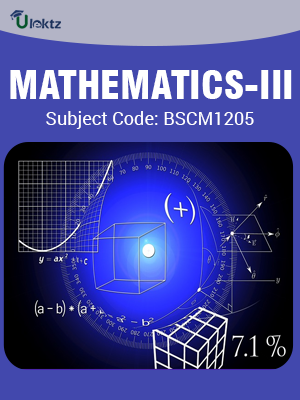•My WalletMy Order
•My Profile
•My Connections
•My Books
•My Videos
•My Tests
•My Calender
•My Messages
•My Shopping Cart
•My Orders
•Account Settings
•Help

# Book Details# Mathematics-III

 Course Code : BSCM1205 Author : uLektz University : Biju Patnaik University of Technology (BPUT) Regulation : 2010 Categories : Computer Science Format :ePUB3 (DRM Protected) Type : eBook

FREE

Description :Mathematics-III of BSCM1205 covers the latest syllabus prescribed by Biju Patnaik University of Technology (BPUT) for regulation 2010. Author: uLektz, Published by uLektz Learning Solutions Private Limited.

Note : No printed book. Only ebook. Access eBook using uLektz apps for Android, iOS and Windows Desktop PC.

##### Topics
###### Module-I Partial Differential Equations & Laplace Equations

1.1 Partial differential equation of first order, Linear partial differential equation, Non-linear partial differential equation

1.2 Homogenous and non-homogeneous partial differential equation with constant co-efficient, Cauchy type, Monge’s method

1.3 Second order partial differential equation

1.4 The vibrating string, the wave equation and its solution, the heat equation and its solution, Two dimensional wave equation and its solution

1.5 Laplace equation in polar, cylindrical and spherical coordinates, potential

###### Module-II Complex Analysis

2.1 Complex Analysis: Analytic function, Cauchy-Riemann equations

2.2 Laplace equation, Conformal mapping

2.3 Complex integration: Line integral in the complex plane, Cauchy’s integral theorem, Cauchy’s integral formula, Derivatives of analytic functions

###### Module–III Power Series, Taylor’s series &Laurent’s series

3.1 Power Series, Taylor’s series, Laurent’s series

3.2 Singularities and zeros

3.3 Residue integration method, evaluation of real integrals